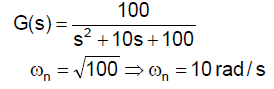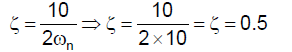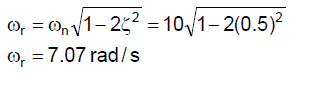Courses

# Control System - MCQ Test 2

## 20 Questions MCQ Test GATE Electrical Engineering (EE) 2022 Mock Test Series | Control System - MCQ Test 2

Description
This mock test of Control System - MCQ Test 2 for GATE helps you for every GATE entrance exam. This contains 20 Multiple Choice Questions for GATE Control System - MCQ Test 2 (mcq) to study with solutions a complete question bank. The solved questions answers in this Control System - MCQ Test 2 quiz give you a good mix of easy questions and tough questions. GATE students definitely take this Control System - MCQ Test 2 exercise for a better result in the exam. You can find other Control System - MCQ Test 2 extra questions, long questions & short questions for GATE on EduRev as well by searching above.
QUESTION: 1

Solution:
QUESTION: 2

### The transfer function of a second order system is given as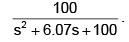The peak (A) value of the output for unit  step input is

Solution: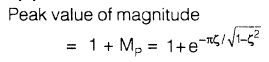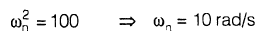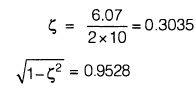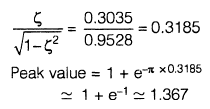QUESTION: 3

### If the resonant peak of the system is B, then the value of B/A is

Solution: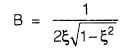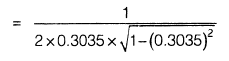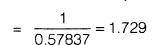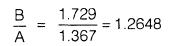QUESTION: 4

A portion of the polar plot of an open-loop transfer function is shown in the given figure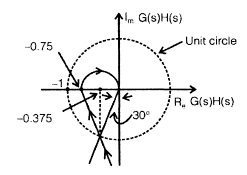The phase margin and gain margin will be respectively

Solution:

Phase margin = 90° − 30° = 60° Gain margin = 1/0.75

QUESTION: 5

The open-loop transfer function of a unity negative feedback system is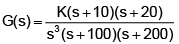The polar plot of the system will be

Solution: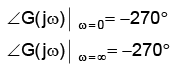The polar plot intersects with the negative real axis as in making imaginary term of G(jω) to be zero, the solution exists.

QUESTION: 6

The compensator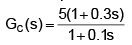would provide a maximum phase shift of

Solution:

Maximum phase shift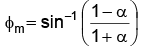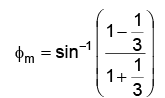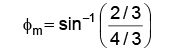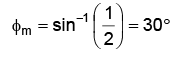QUESTION: 7

A step input is applied to a system with the transfer function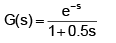The output response will be

Solution: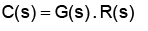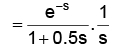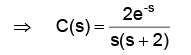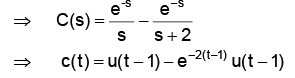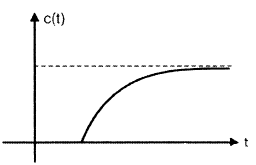QUESTION: 8

Which one of the following characteristic equations of result in the stable operation of the feedback system?

Solution:

For stable operation, all coefficients of the characteristic equation should be real and have the same sign and none of the coefficients should be zero.

QUESTION: 9

Consider the following statements:
Routh-Hurwitz criterion gives
1. absolute stability
2. the number of roots lying on the right half of the s-plane
3. the gain margin and phase margin
Which of these statements are correct?

Which of these statements are correct?

Solution:

Routh - Hurwitz criterion gives absolute stability and the number of roots lying on the right half of the s-plane but it does not tell about the gain margin and phase margin.

QUESTION: 10

The polar plot (for positive frequencies) for the open-loop transfer function of a unity feedback control system is shown in the given figure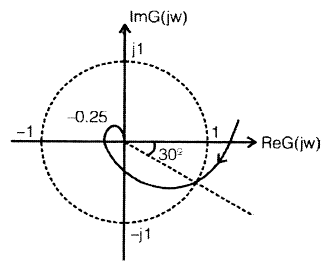The phase margin and the gain margin ofthe system are respectively

Solution: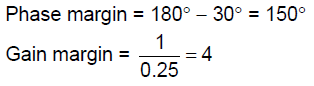QUESTION: 11

For the given network, the maximum phase lead φm of V0 with respect to Vi is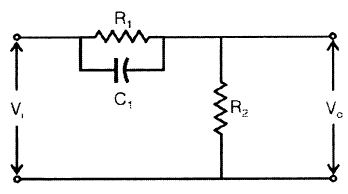Solution: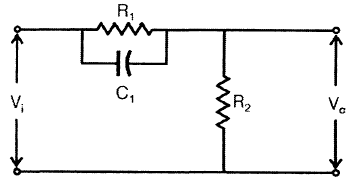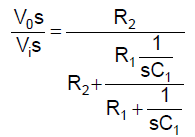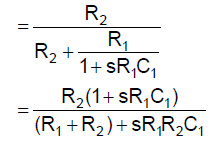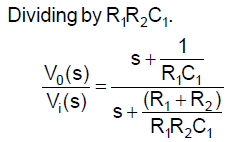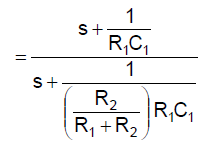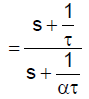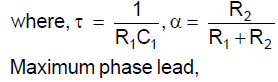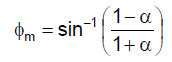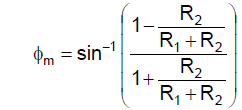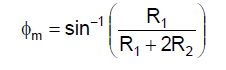QUESTION: 12

For the system dynamics described by differential equationy+3y+2y=u(t) the transfer function of the system represented in controllable canonical form is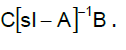The matrix A would be

Solution:

General representation of phase variable representation: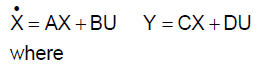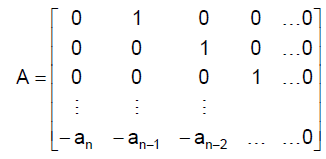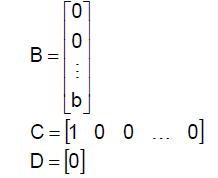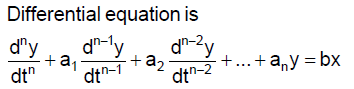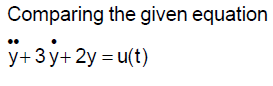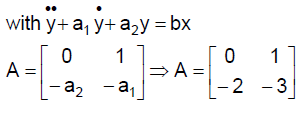QUESTION: 13

For the signal flow diagram shown in the given figure, the transmittance between x2 and x1 is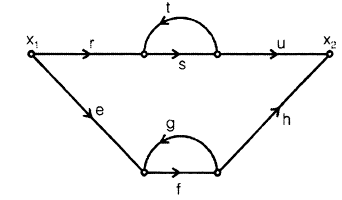Solution: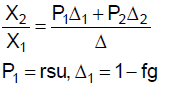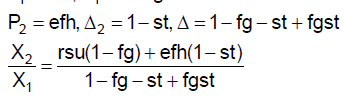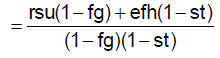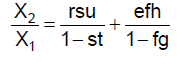QUESTION: 14

Consider the mechanical system shown in the given figure. If the system is set into motion by unit impulse force, the equation of the resulting oscillation will be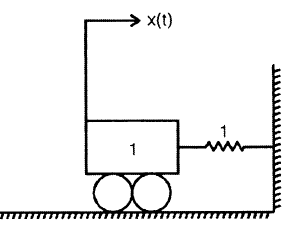Solution: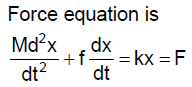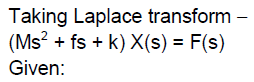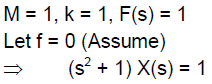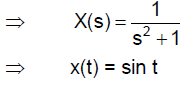QUESTION: 15

Nyquist plot shown in the given figure is for a type

1.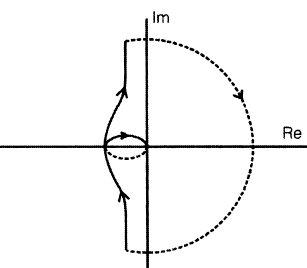Solution: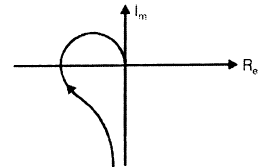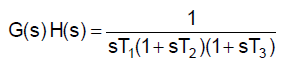(i) When a pole is added at negative real axis, the tail of the plot remains at the same position whereas head of plot shifts by 90° in clockwise direction.

(ii) When a pole is added at origin, both the tail and head of the plot shift by 90° in clockwise direction.

QUESTION: 16

An open loop transfer function of a unity feedback control system has two finite zeros, two poles at origin and two pairs of complex conjugate poles. The slope of high frequency asymptote in Bode magnitude plot will be

Solution:

No. of poles, n = 6
No. of zeros, m = 2
Slope of high frequency asymptote
= −20 (n−m)
= −20 (6−2)
= −80 dB/decade

QUESTION: 17

Match List-I (Pole-zero plot of linear control system) with
List-II (Responses of the system) and select the correct answer:
Codes: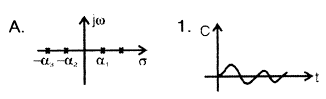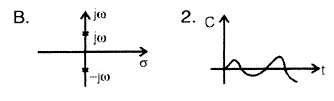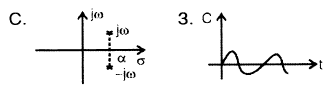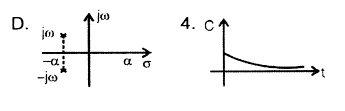Codes:

A    B    C  D

Solution:
QUESTION: 18

The unit impulse response of a system having transfer function K/(s + α) is shown below. The value of α is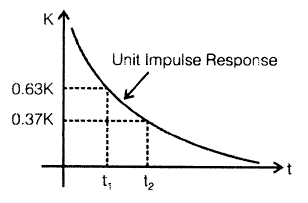Solution: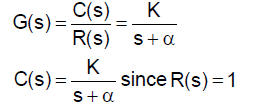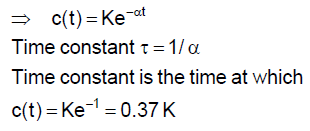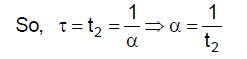QUESTION: 19

The transfer function of a phase lead network as shown in the figure below is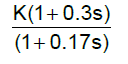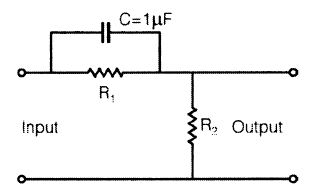The values of R1 and R2 are respectively

Solution: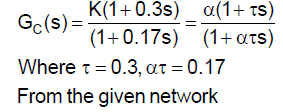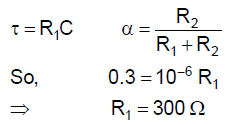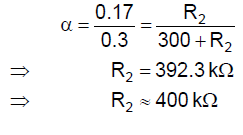QUESTION: 20

The forward path transfer function of a unity feedback system is given by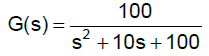The frequency response of this system will exhibit the resonance peak at

Solution: